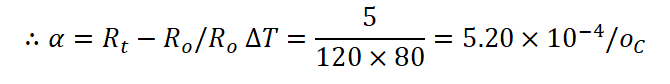# Critical Temperature MCQs with Answers

We know that when temperature of metallic conductor increases, its resistance increases due to increase in inter atomic vibrations of electrons with cores of neighbouring atom. The formula for resistance of conductor when due to rise in temperature (T0C) is given as,

Rt = Ro (1 – αT)

Where, α = temperature coefficient of resistance, T = rise in temperature.

Now if we go reverse means, the temperature of material of conductor is decreases, inter atomic vibrations will decrease, which results the fall in resistance of conductor. If we cool the given conductor up to certain temperature so that its resistance reduces to zero, the corresponding temperature is known as critical temperature of material of conductor. At critical temperature the resistance of conductor becomes zero. If we further cool the conductor below its critical temperature, it’s become superconductor and the phenomenon is known as superconductivity.

Critical temperature of metal/conductor is defined as the temperature at which the resistivity of metal becomes zero.

Q.1) Resistance of metal wire………..with increase in temperature.

a) decreases

b) increases

c) remains same

d) can be predicted

Q.2) Critical temperature of material is the temperature at which resistance of material of conductor becomes……….

a) infinitely high

b) very low

c) zero

d) half the original value

Q.3) If metal is cooled below its critical temperature, it acts as………………

a) Mega conductor

b) Superconductor

c) Super resistor

d) Mega Resistor

Q.4) For metals, the value of temperature coefficient of resistance has………..value

a) positive

b) negative

c) either positive of negative

d) none of these

Q.5) Critical temperature for mercury is….

a) 42 K

b) 420 K

c) 4.2 K

d) 42 K

Q.6) Select the statement which is ‘not correct’ in case of change in temperature of metal.

a) Inter atomic vibrations in metal increase with increase in temperature.

b) Resistance of metal becomes zero at critical temperature.

c) Below critical temperature the resistance of metal shows sharp rise.

d) All above statements are not correct for the metals.

Q.7) Which among the following materials, value of temperature coefficient of resistance is negative?

a) Insulators

b) Semiconductors

c) Conductors

d) All of these

Q.8) The value of temperature coefficient of resistance of wire of resistance 120 Ω and increase in resistance of wire when heated from 200C to 1000C is 5 Ω

a) 2.20 × 10-4/oC

b) 3.20 × 10-4/oC

c) 4.20 × 10-4/oC

d) 5.20 × 10-4/oCQ.9) Which among the following is correct for conductors having temperature equal to its critical temperature?

a) conductor acquire very high resistance

b) resistance of conductors reduces to zero

c) conductors can acts as superconductor

d) both ‘b’ and ‘c’

Q.1) b) increases

Q.2) c) zero

Q.3) b) Superconductor

Q.4) a) positive

Q.5) c) 4.2 K

Q.6) c)Below critical temperature the resistance of metal shows sharp rise.

Q.7) b) Semiconductors

Q.8) d) 5.20 × 10-4/oC

Q.9) d) both ‘b’ and ‘c’

Updated: July 15, 2021 — 12:50 am